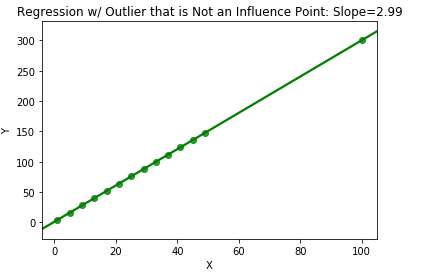# What is the difference between outliers, high leverage points, and high influence points?

Outlier is a general term for an observation that is far away from most other data points. Outliers are often identified subjectively, but common heuristics include points beyond 1.5 interquartile ranges from the first and third quartiles, or those a certain number of standard deviations beyond the mean.

A high leverage point specifically refers to an observation in which the value of a predictor is considered to be extreme in the feature space. Thus, leverage points can be considered outliers in the context of the predictor space (X), whereas in regression analysis, outliers usually specifically refer to the scope of the target variable (Y).

High influence points are observations that most influence, hence the name, the shape of the regression equation. If an observation is a high influence point, the slope of the regression line would change significantly if that one point was removed from the data set. While outliers and leverage points are defined in the context of the target and feature space, respectively, influence points are found in the (X,Y) space, as for an observation to have a large sway over the regression equation, it requires information from both the X and Y spaces. In the case of a single predictor, a high influence point would be one that either bends the line noticeably upward or downward, thus having an undue effect on the estimate of the slope. The effect of an influence point is shown in the dummy data below, where the presence of a single observation reduces the magnitude of the slope of the regression by almost ⅓. However, in the third graph, an observation that is both a leverage point (in X) and outlier (in Y) is not a high influence point because it has little effect on the slope of the regression despite being isolated in both the X and Y space.The most common diagnostic for detecting influence points is Cook’s Distance, and points that have large Cook values might need to be investigated further. In the process of data exploration, it is important to verify the quality of the data being captured, as it is often the case that these points are due to issues in the underlying collection of the data.

Find out all the ways
that you can